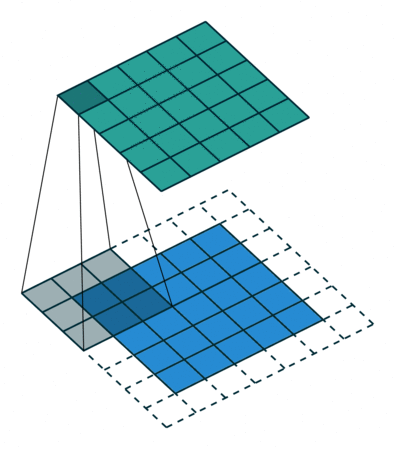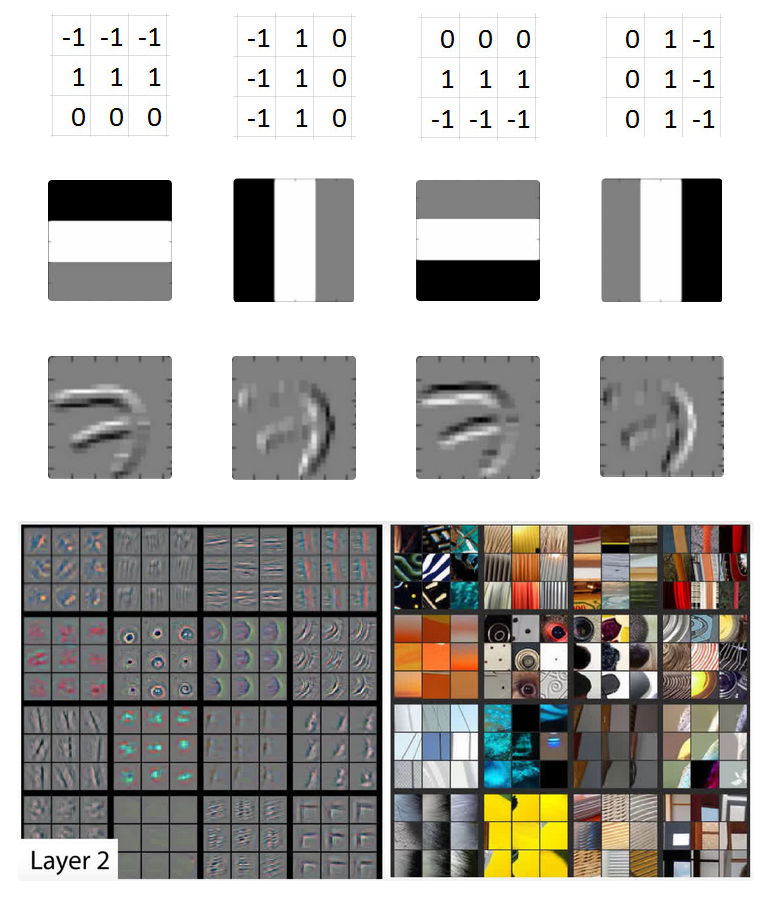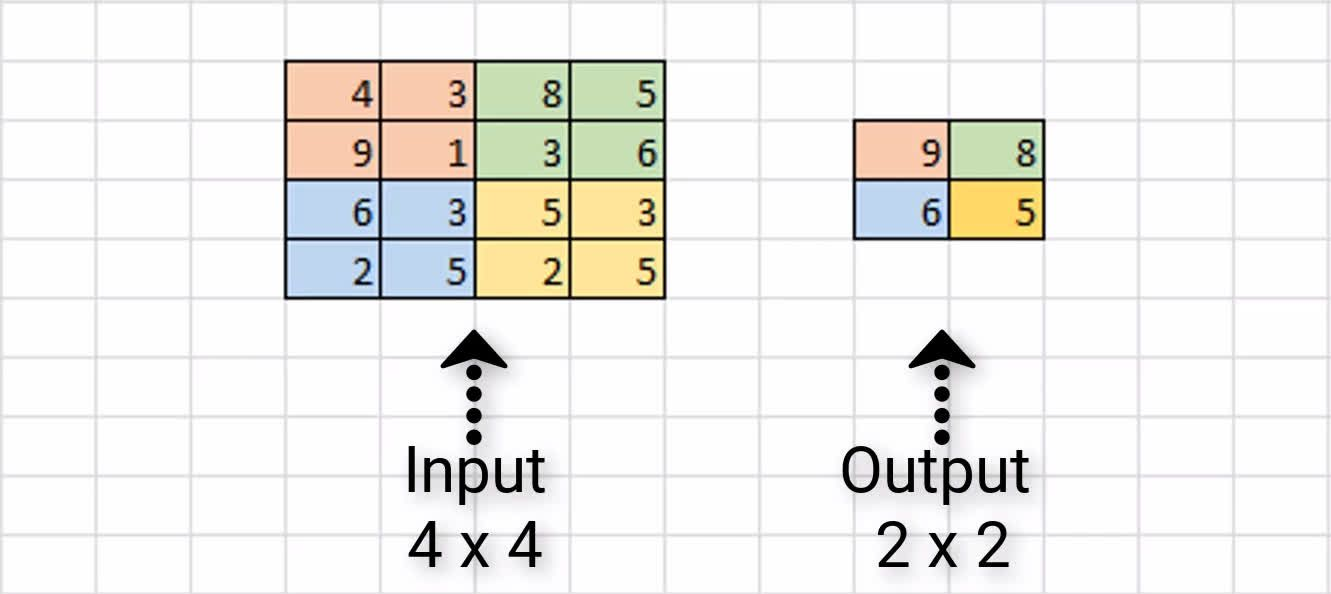# Convolutional networks

Caution! This article is 3 years old. It may be obsolete or show old techniques. It may also still be relevant, and you may find it useful! So it has been marked as deprecated, just in case.

A convolutional neural network / CNN / ConvNet is an artificial neural network that detects patterns in images using filters. They are mostly used for analyzing images for computer vision tasks.

The inputs to convolutional layers are called input channels, and the outputs are called output channels.

The transformation is called a convolution operation in the deep learning community, and cross-correlation mathematically. A convolution operation maps an input to an output using a filter and a sliding window.

## Filters

We need to specify the number of filters each layer should have. The number of filters determines the number of output channels.

These filters are what detect the patterns. Patterns can be edges, shapes, textures, curves, objects, colors, etc. in the first layers. The deeper the network goes, the more sophisticated the filters become.Animation of a 3x3 CNN filter (gray) sliding through the input channel in the bottom (blue) and the resulting output channel at the top (green).

The convolution of a filter and each subset of the same size in the input channel is calculated as the summation of the element-wise products.

For example, for a 3x3 filter:

``````
| a11 a12 a13 |           | b11 b12 b13 |
filter = | a21 a22 a23 |  subset = | b21 b22 b23 |
| a31 a32 a33 |           | b31 b32 b33 |

product = a11 b11 + a12 b12 + ... + a33 b33
``````

Because the filter is 3x3, the resulting output channel will be smaller by a margin of 1 pixel on all sides.

Sometimes this is called dot product since it is an inner product (generalization of the dot product). Other names are "Frobenius inner product" or "summation of the Hadamard product".The four filters detect horizontal and vertical edges in one sample of the MNIST dataset representing the number 7. The "Layer 2" image shows edge detection examples from several images in the first layers of a CNN.

In the past, computer vision experts would develop filters manually (for example, the Sobel filter). Today, pattern detectors are derived automatically by the network as it learns. The filter values start out with random values, and the values change as the network learns during training.

Use this interactive demonstration to gain a better understanding of convolutions, or Francois Chollet post "How CNNs see the world".

Convolutions reduce output channel dimensions by `(n - f + 1)(m - g + 1)`, where `n x m` are the dimensions of the input and `f x g` are the dimension of the filter.

This is a problem when there is meaningful information around the edges of the image. The solution is to use zero padding, a technique where we add a border of pixels with value zero around the edges of the input image. This allows us to preserve the original input size as convolutions are applied.

`valid` No padding Dimensions reduce
`same` Zeros around the edges Dimensions stay the same

In Keras we specify the filter size with `kernel_size` and the padding with `padding`:

``````
model = Sequential([
Dense(16, input_shape=(20,20,3), activation='relu'),
Conv2D(32, kernel_size=(3,3), activation='relu', padding='valid'),
Dense(2, activation='softmax')
])
``````

Output goes from `(20, 20)` to `(18, 18)`:

``````
model.summary()
_________________________________________________________________
Layer (type)                 Output Shape              Param #
=================================================================
dense_2 (Dense)              (None, 20, 20, 16)        64
_________________________________________________________________
conv2d_1 (Conv2D)            (None, 18, 18, 32)        4640
_________________________________________________________________
dense_3 (Dense)              (None, 2)                 16386
``````

## Max Pooling

Max pooling is added after a convolutional layer, and it reduces the number of pixels in the output from the previous convolutional layer. It's used to reduce computational load (smaller image -> less parameters) and reduce over-fitting.

We define the size `n x m` of the "pool", and a stride, or how many pixels we will move to find the next region. Every pixel of the output is calculated as the maximum of the values in the corresponding region of the image. With a pool of 2x2 and a stride of 2, the output will be reduced to half the size of the input.

Average pooling is where we take the average value from each region rather than the maximum. Currently, max pooling is used vastly more.Maximum pooling applied to a 4x4 image, with a 2x2 pool and stride 2, produces an output that is 2x2.

In Keras, there is a specific layer for max pooling, where we can specify both the size of the pool and the stride. They usually have no padding:

``````
MaxPooling2D(pool_size=(2, 2), strides=2, padding='valid')
``````

There are also `MaxPooling1D` and `MaxPooling3D`.